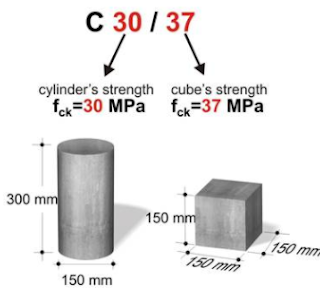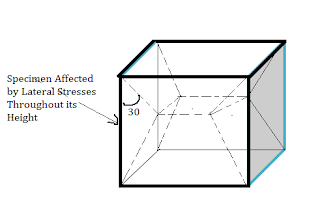# What is the difference between Concrete Cube and Cylinder Strength test?

### The compressive strength test of concrete is conducted either on a concrete cube or cylindrical specimen. It has been observed that the final compressive strength test value is greater for a cubical specimen compared to a cylindrical specimen, given that both have the same concrete mix.Difference between Concrete Cube and Cylinder Strength test

The main reason behind this variation in strength is the length to diameter ratios of cylinder and concrete specimen.

• The length to diameter ratio of a cylindrical specimen used for the compressive strength test is 2: 1, while that for a cube is 1:1. Hence, cubes are found stronger than cylinders.
• Compared to the cylinder, the cube provides more contact area for the upper platen in the compressive testing machine, giving more confinement.
• Cubes are confined more by the platens in the compressive testing machine, this would demand higher stress for the cube to undergo failure than a cylinder. Hence the test value shows higher compressive strength for cubes.
The steel plates are placed above and below the specimen (cube/ cylinder) before the specimen is loaded. There is no kind of lateral expansion carried out in the steel plates when compared to the concrete specimen. This means that the steel will restrain the tendency of concrete to expand in the lateral direction. This restrains the lateral expansion of the concrete specimen by the platens. This is prevalent in the concrete element of the specimen which is near the end of the plate. As a result of this, frictional stress arises between the plate and the specimen, in addition to the compressive stress due to applied compressive load.

Hence under normal conditions, an element within the specimen will undergo a combination of “shear stress”. This is because of the

• Friction between the plates and the specimen – Stress 1
• Compressive Load due to the loading action - Stress 2
It is observed that the combination of Stress 1 and Stress 2 will result in axial load failure. This will result in providing a compressive strength value that is higher.

It has been observed that the restraining effect due to friction between the specimen and the platens extends over the entire height of the cube. But in the case of the cylinder, it remains unaffected.  The activity is explained in the figure-3 and figure-4.be and Cylinder Strength RelationFig.3. Region Affected by Lateral Stresses in CylinderFig.4. Region Affected by Lateral Stresses in Cubes

Read On : What is Target Mean Strength?

It is observed that the restraining effect due to friction between the specimen and the platens extends over the entire height of the cube. But in the case of the cylinder, it remains unaffected.  The activity is explained in the figure-3 and figure-4. The cylinders fail by shear at 60 degrees and cubes at 45 degrees to the horizontal.

Hence, it is clear that the total stress that will be created in the cube will be higher compared with the cylinder specimen. This will result in a higher value of the compressive strength in cubes than in the cylinder even by employing the same concrete mix. Hence their relation can be given as:

Compressive Strength of cylinder = 0.8 x ( Compressive Strength of Cube )

Given the same concrete mix is used in both sample